Get instant live expert help with Excel or Google Sheets“My Excelchat expert helped me in less than 20 minutes, saving me what would have been 5 hours of work!”

#### Post your problem and you’ll get expert help in seconds.

Your message must be at least 40 characters
Our professional experts are available now. Your privacy is guaranteed.

# Using the Logical Operator Not Equal To In Excel

We can use the “Not equal to” comparison operator in Excel to check if two values are not equal to each other. In Excel, the symbol for not equal to is <>. When we check two values with the not equal to formula, our results will be Boolean values which are either True or False. In this tutorial, we will explore the ways to use the Not Equal to Boolean operator in Excel.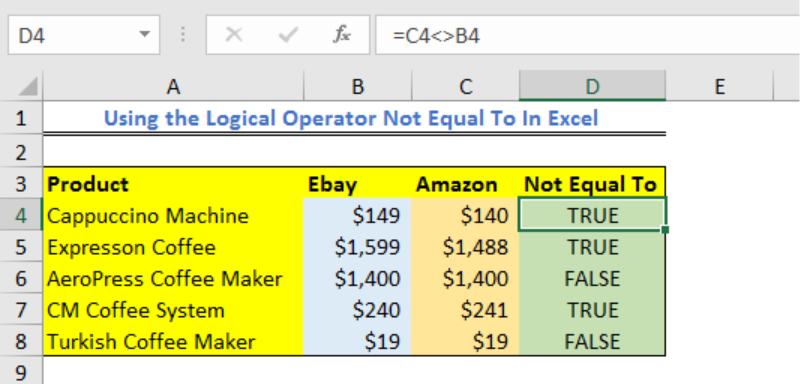Figure 1 – Not equal sign in Excel

## Using the “Not Equal to” to test numeric values and text values

We will prepare a data table and then test values from our data table using the <> symbol.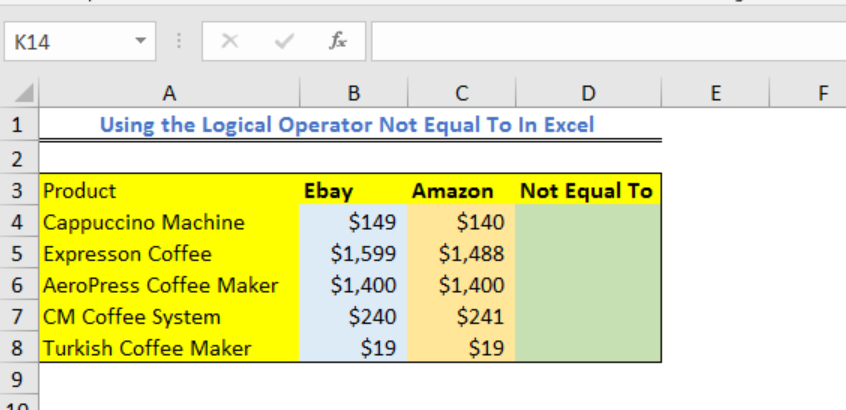Figure 2 – Data for showing the excel not equal formula

• In Cell D4, we will enter the formula below and press OK
`=C4<>B4`
• Our result will be displayed as either TRUE or FALSE.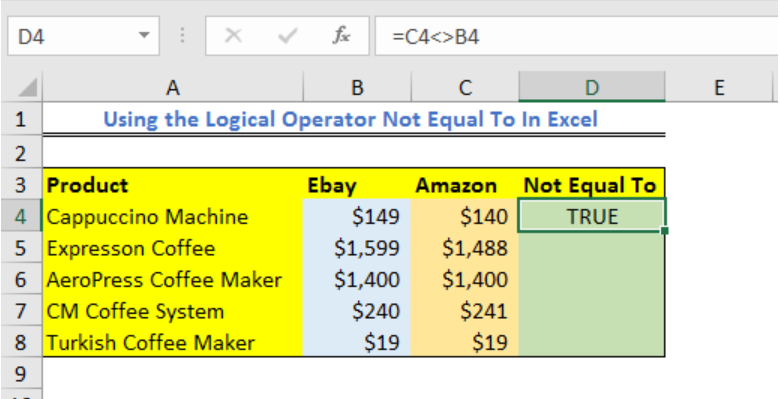Figure 3 – Not equal to in excel

• If we drag down the formula into other cells in Column C, we will have: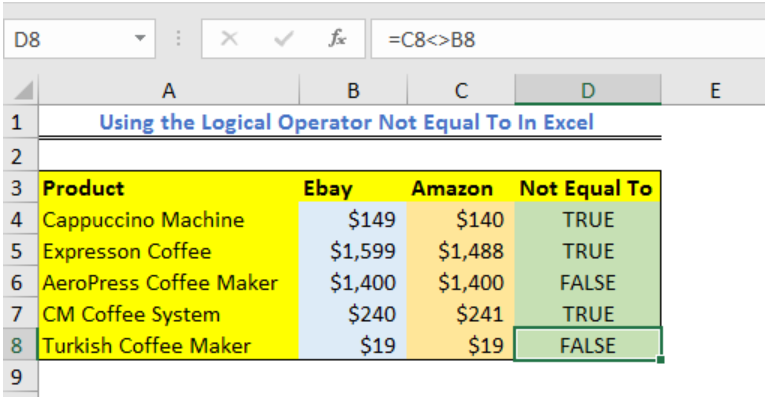Figure 4 – Does not equal in excel

## Using a “Not Equal To” in Excel IF Formula

We can write IF Statements with the not equal to operator to show specific results when particular conditions are met or not.

• Again, we will prepare a data table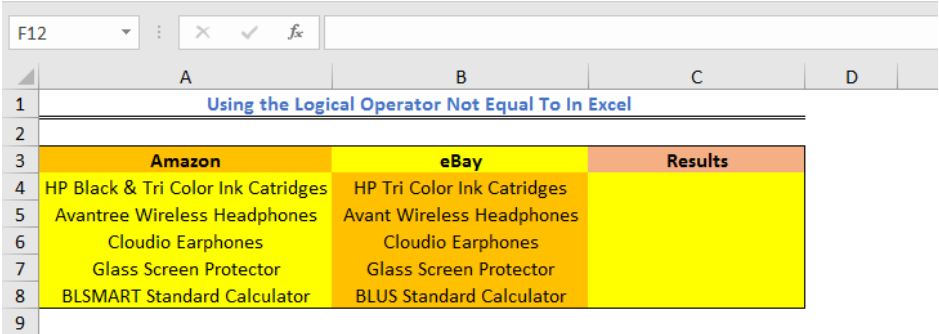Figure 5 – Does not equal in excel

• In Cell C4, we will insert the formula and press the enter key
`=IF(A4<>B4,"Product Not Matching", "Product Matching")`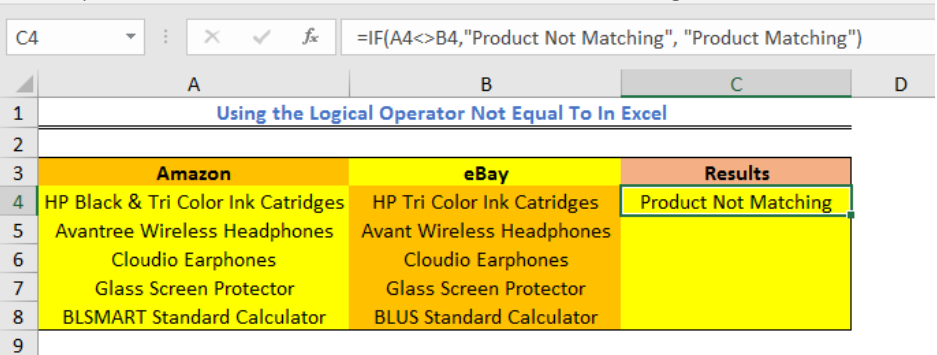Figure 6 – How to do not equal in excel

• We will copy down the formula into other cells.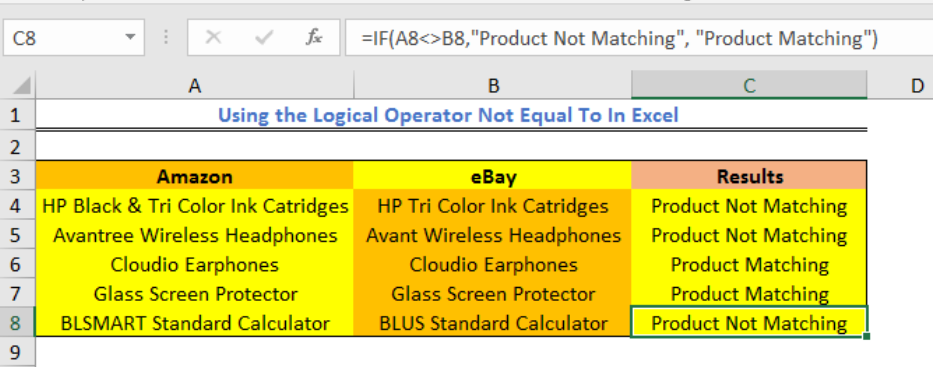Figure 7 – Not equal to symbol in excel

## Using the Not Equal to in Excel COUNTIF formula

We can use the not equal to operator to count the number of cells that contain values not equal to a particular value. In this section, we will use the COUNTIF function and the not equal to operators to count other items in our list except the specified item.

• We will prepare a data table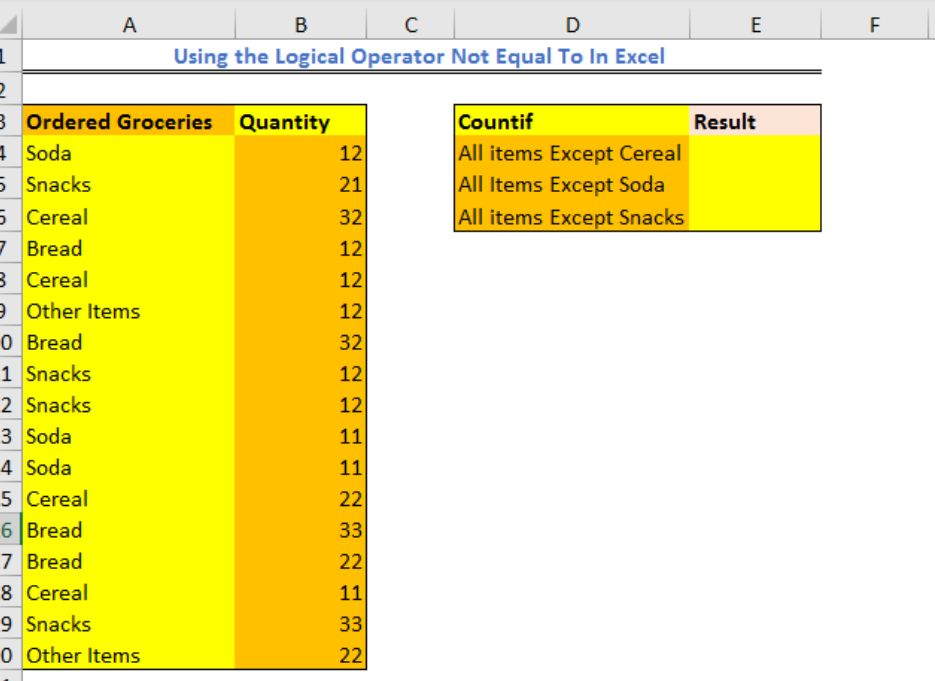Figure 8 – Boolean operators in excel

• To count all items except Cereal. In Cell E4, we will enter the formula below and press the enter key.
`=COUNTIF(A4:A20,"<>Cereal")`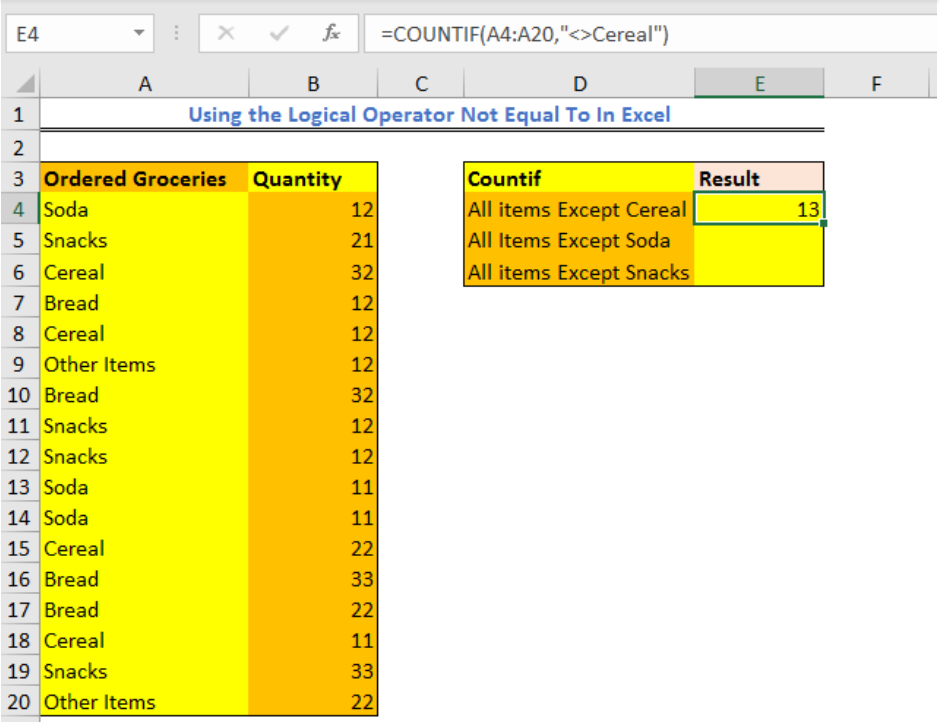Figure 9 – comparison operators in excel

• To Count all other items except Soda, we will click on Cell E5, and enter the formula below.
`=COUNTIF(A4:A20,"<>Soda")`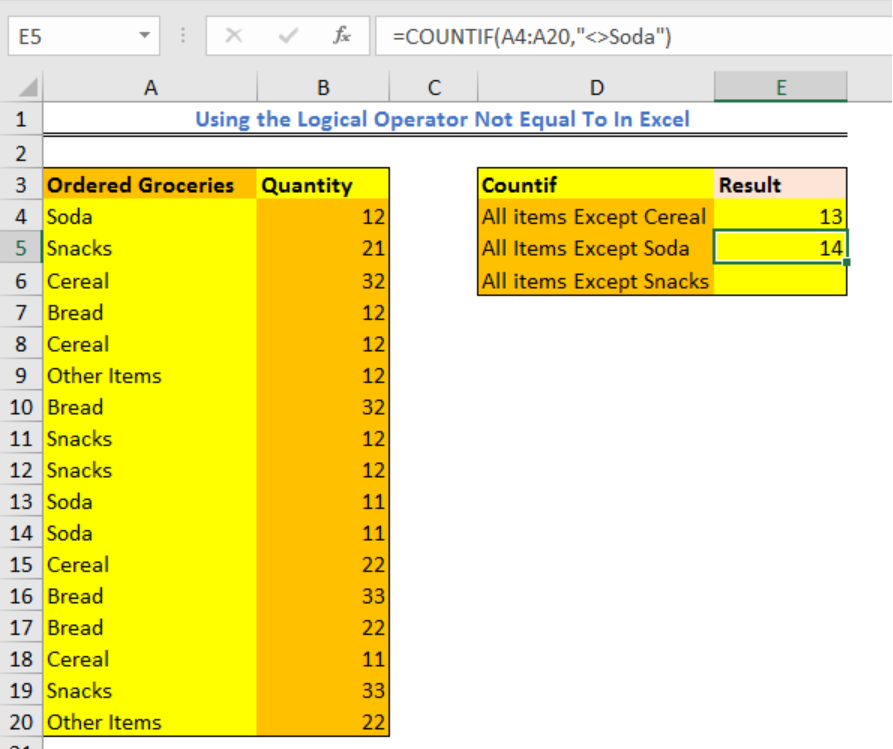Figure 10 – Does not equal in excel

1. To Count all items except Snacks, we will click on Cell E6, and enter the formula below
`=COUNTIF(A4:A20,"<>Snacks")`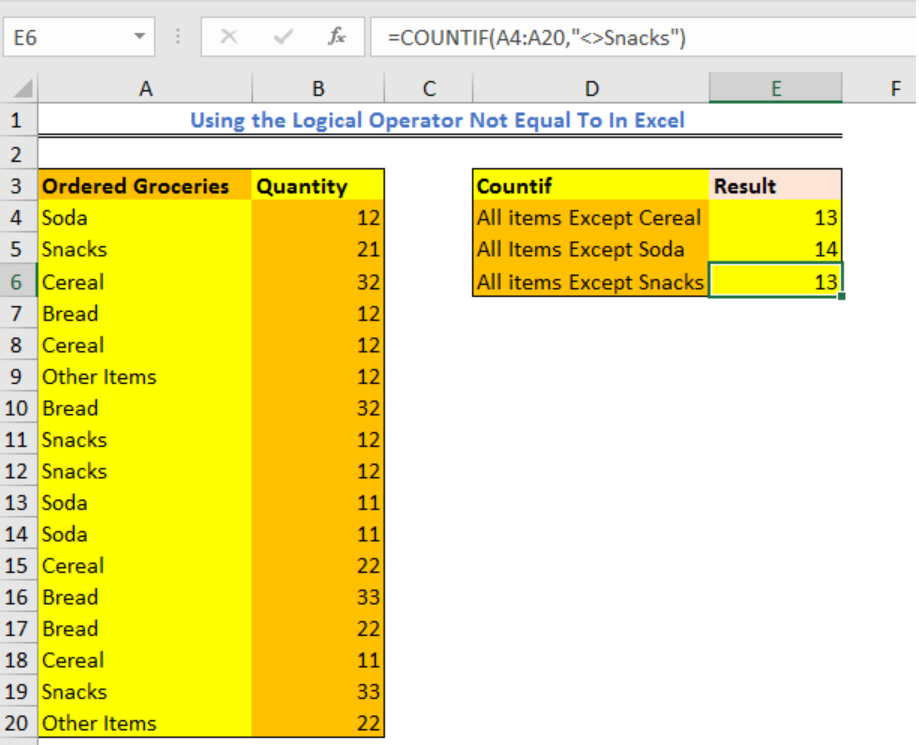Figure 11 – Boolean operator in excel

## Instant Connection to an Excel Expert

Most of the time, the problem you will need to solve will be more complex than a simple application of a formula or function. If you want to save hours of research and frustration, try our live Excelchat service! Our Excel Experts are available 24/7 to answer any Excel question you may have. We guarantee a connection within 30 seconds and a customized solution within 20 minutes.